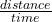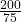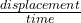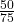## A runner travels 2.5 laps around a circular track in a time of 75 sec. The diameter of the track is 50 m and its circumference is 120 m. Fin

Question

A runner travels 2.5 laps around a circular track in a time of 75 sec. The diameter of the track is 50 m and its circumference is 120 m. Find (a) the average speed of the runner and (b) the magnitude of the runner’s average velocity. Average speed depends on the total distance traveled, whereas average velocity depends on the displacement at the end of the particular journey.

in progress 0
2 months 2021-07-31T23:51:36+00:00 1 Answers 3 views 0

2.67m/s

0.67m/s

Explanation:

Given parameters:

Number of laps run = 2.5laps

time taken  = 75 sec

Diameter of track  = 50m

Circumference  = 120m

Unknown:

Average speed of runner  = ?

Average velocity of runner  = ?

Solution:

Average speed depends on the total distance traveled,  it is the rate of change of distance with time. It covers the full extent of the path;

Average speed  =distance = circumference

Average speed  == 2.67m/s

Average velocity depends on the displacement at the end of the particular journey. The displacement deals with the length of path from start to finish in a specific direction.

The diameter is the same as displacement here.

Average velocity  === 0.67m/s